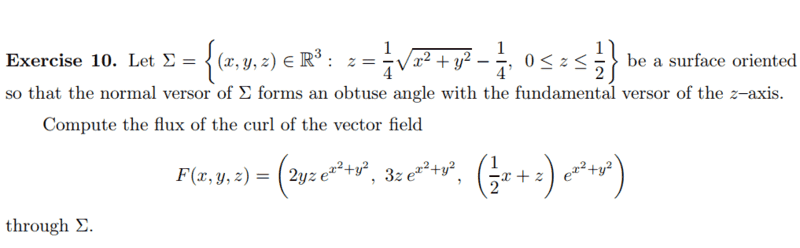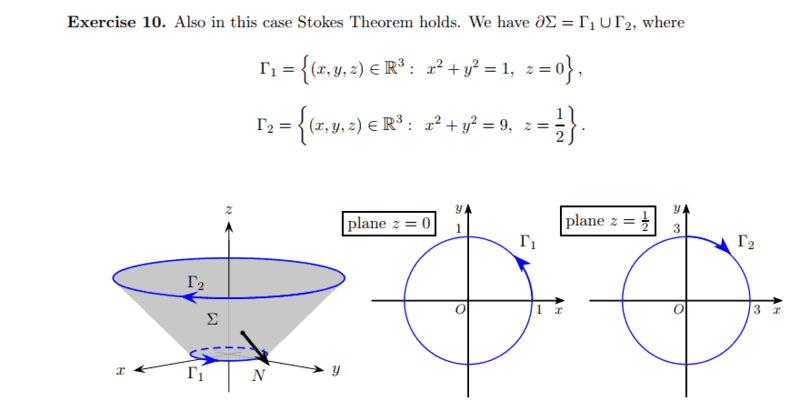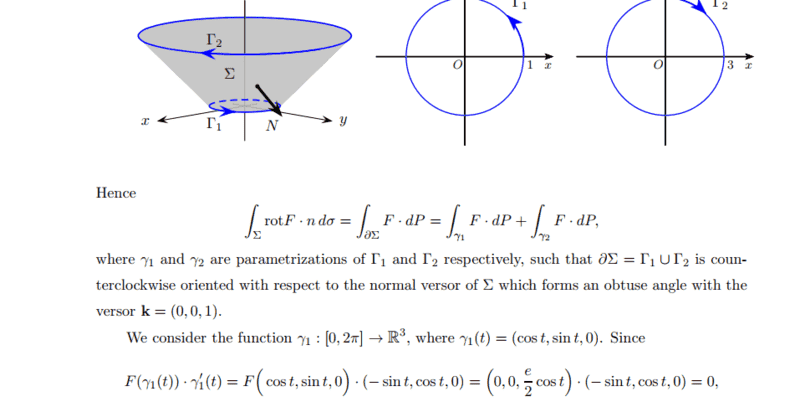# Problem with the positive orientation of a surface (Stokes' theorem)

Amaelle
Homework Statement:
look at the image
Relevant Equations:
stockes theorem
Greetingsthe solution is the following which I understandI do understand why the current orientation of the Path is positive regarding to stocks (the surface should remain to the left) but I don´t understand why the current N vector of the surface is positive regarding stockes theorem

thank you!

•Delta2

Mentor
Homework Statement:: look at the image
Relevant Equations:: stockes theorem

Greetings
View attachment 302035
the solution is the following which I understand
View attachment 302036

View attachment 302037

I do understand why the current orientation of the Path is positive regarding to stocks (the surface should remain to the left) but I don´t understand why the current N vector of the surface is positive regarding stockes theorem

thank you!
First off, the name is Stokes, not stockes and not stocks. The theorem is Stokes' (or Stokes's) Theorem, AKA Kelvin-Stokes Theorem.

Regarding your question, "I don´t understand why the current N vector of the surface is positive" -- I'm not sure what you mean by N being positive. Numbers can be positive or negative or zero, but vectors aren't comparable to numbers.

The problem statement, "the normal versor of ##\Sigma## forms an obtuse angle with the fundamental versor of the z-axis." I interpret this to mean that N is the vector that makes an obtuse (> 90°) angle with the positive z-axis. That means that N points outward and down, as shown in the drawing. A normal vector that pointed inward and up would make an acute angle with the positive z-axis.

Homework Helper
Gold Member
What I find really interesting in this problem is the surface and its boundary. More specifically the boundary ##\partial\Sigma## consists of two closed curves and not just one.

•Amaelle
Staff Emeritus
Homework Helper
Gold Member
What I find really interesting in this problem is the surface and its boundary. More specifically the boundary ##\partial\Sigma## consists of two closed curves and not just one.
If you want a single closed curve you can add and subtract a curve going from one to the other.

Homework Helper
Gold Member
I think the problem usually with surface is if the normal vector will be taken positive as inwards or outwards. For this particular surface, if it is taken positive as inwards, then it makes an acute angle with z-axis, if it is taken positive as outwards, then it makes an obtuse angle with the z-axis. But ok now I see @Mark44 already did a similar comment.

Btw haven't heard the term "versor" before, from the context I think its synonym to "unit vector".

Mentor
Btw haven't heard the term "versor" before, from the context I think its synonym to "unit vector".
I had to look it up as well. Apparently it was coined by William Rowan Hamilton. Some sources make the claim that Hamilton also coined the word vector to describe the (i, j, k) part of a quaternion. Other sources cite earlier uses of the word "vector."

•Delta2
Amaelle
in the case of one simple surface the direction of the path determine the orientation of the surface with the right hand rule, in this case we have two borders if one gives us a surface with an orientation that goes with N and the second with one negative with N I hope you got my point

Staff Emeritus
Homework Helper
Gold Member
in the case of one simple surface the direction of the path determine the orientation of the surface with the right hand rule, in this case we have two borders if one gives us a surface with an orientation that goes with N and the second with one negative with N I hope you got my point
If that is the case then the curves do not form the directed boundary of the surface and you cannot apply the curl theorem.

Edit: … and note that this is not the case here. Both curves indicate the same normal direction.

Staff Emeritus
Homework Helper
Gold Member
The theorem is Stokes' (or Stokes's) Theorem, AKA Kelvin-Stokes Theorem.
AKA the curl theorem. I prefer this as it avoids confusion with Stokes’ theorem (aka Stokes-Cartan theorem) of which it is a special case.

Amaelle
If that is the case then the curves do not form the directed boundary of the surface and you cannot apply the curl theorem.

Edit: … and note that this is not the case here. Both curves indicate the same normal direction.
thank you
you said it´s not the case herewhen I apply the right hand rule on curve1 it goes up down when I apply it to curve 2, is there soemthing I'm missing?

Staff Emeritus
Homework Helper
Gold Member
is there soemthing I'm missing?
Yes, you are applying the right hand rule wrong. You are applying it as if the surface was a disc in the middle of the curve - it is not.

The correct way to apply the right hand rule is to think about grabbing the curve with your right hand with the the thumb on the side the curve is directed towards. Then the normal of the surface is in the direction your other fingers point.

•Amaelle
Amaelle
thanks a million for all of you ! you made the pain easier to bear :)

•Delta2
Homework Helper
Gold Member
thanks a million for all of you ! you made the pain easier to bear :)
Its the pain of gaining new knowledge e hehe

No pain no gain!

•Amaelle
Staff Emeritus
Pain is weakness leaving the body.••Pain is weakness leaving the body.